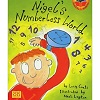Resource Title search

Text Search of NRICH resource titles

Or find resource begining with: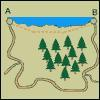N Is a Number

Age 11 to 14 Challenge Level:

N people visit their friends staying N kilometres along the coast. Some walk along the cliff path at N km an hour, the rest go by car. How long is the road?N000ughty Thoughts

Age 14 to 16 Challenge Level:

How many noughts are at the end of these giant numbers?Name That Graph

Age 16 to 18 Challenge Level:

How can you work out the equation of a parabola just by looking at key features of its graph?Name That Graph Again

Age 16 to 18 Challenge Level:

If you know some information about a parabola, can you work out its equation?Name That Triangle!

Age 7 to 11 Challenge Level:

Can you sketch triangles that fit in the cells in this grid? Which ones are impossible? How do you know?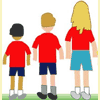Name the Children

Age 5 to 7 Challenge Level:

Can you find out in which order the children are standing in this line?Named Products

Age 11 to 14 Short Challenge Level:

Can you find the solution to this equation? Each of the different letters stands for a different number.Napier's Bones

Age 7 to 11 Challenge Level:

The Scot, John Napier, invented these strips about 400 years ago to help calculate multiplication and division. Can you work out how to use Napier's bones to find the answer to these multiplications?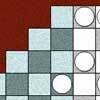Napier's Location Arithmetic

Age 14 to 16 Challenge Level:

Have you seen this way of doing multiplication ?Napkin

Age 14 to 16 Challenge Level:

A napkin is folded so that a corner coincides with the midpoint of an opposite edge . Investigate the three triangles formed .Napoleon's Hat

Age 16 to 18 Challenge Level:

Three equilateral triangles ABC, AYX and XZB are drawn with the point X a moveable point on AB. The points P, Q and R are the centres of the three triangles. What can you say about triangle PQR?Napoleon's Theorem

Age 14 to 18 Challenge Level:

Triangle ABC has equilateral triangles drawn on its edges. Points P, Q and R are the centres of the equilateral triangles. What can you prove about the triangle PQR?National Education Union - Celebrating Education

Age 7 to 16

Resources to accompany Charlie's NRICH workshop at NEU's Celebrating Education Conference.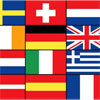National Flags

Age 7 to 11 Challenge Level:

This problem explores the shapes and symmetries in some national flags.National Young Mathematicians' Award Activities

Age 5 to 11 Challenge Level:

The activities on this page are based on those written for the National Young Mathematicians' Award.Natural Born Mathematicians

Age 5 to 7

Helen Joyce interviews the neuropsychologist Brian Butterworth whose research has shown that we are all born with a "built-in" sense of cardinal number.Natural Shapes

Age 14 to 16 Challenge Level:

How does shape relate to function in the natural world?Natural Sum

Age 14 to 16 Challenge Level:

The picture illustrates the sum 1 + 2 + 3 + 4 = (4 x 5)/2. Prove the general formula for the sum of the first n natural numbers and the formula for the sum of the cubes of the first n natural. . . .Near 10

Age 14 to 16 Short Challenge Level:

10 must remain within easy reach...Negative Dice

Age 11 to 14 Short Challenge Level:

If the odd numbers on two dice are made negative, which of the totals cannot be achieved?Negative Dice Poster

Age 7 to 11 Challenge Level:

Negative Dice Poster - September 2007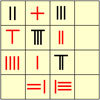Negative Numbers

Age 11 to 14

A brief history of negative numbers throughout the agesNegative Power

Age 14 to 16 Challenge Level:

What does this number mean ? Which order of 1, 2, 3 and 4 makes the highest value ? Which makes the lowest ?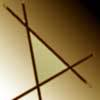Negatively Triangular

Age 14 to 16 Challenge Level:

How many intersections do you expect from four straight lines ? Which three lines enclose a triangle with negative co-ordinates for every point ?Neighbours

Age 7 to 11 Challenge Level:

In a square in which the houses are evenly spaced, numbers 3 and 10 are opposite each other. What is the smallest and what is the largest possible number of houses in the square?Nested Square Roots

Age 14 to 16 Short Challenge Level:

Can you find the value of this expression, which contains infinitely nested square roots?Nested Squares

Age 11 to 14 Short Challenge Level:

What proportion of the diagram is shaded?Nested Surds

Age 16 to 18 Challenge Level:

Can you find values that make these surd statements true?Net Profit

Age 11 to 14 Short Challenge Level:

The diagram shows the net of a cube. Which edge meets the edge X when the net is folded to form the cube?Net Result

Age 11 to 14 Short Challenge Level:

The net shown here is cut out and folded to form a cube. Which face is then opposite the face marked X?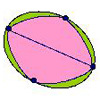Networks and Nodes

Age 7 to 11 Challenge Level:

Without taking your pencil off the paper or going over a line or passing through one of the points twice, can you follow each of the networks?Neural Nets

Age 16 to 18

Find out some of the mathematics behind neural networks.Never Prime

Age 14 to 16 Challenge Level:

If a two digit number has its digits reversed and the smaller of the two numbers is subtracted from the larger, prove the difference can never be prime.New Building Blocks: a Review of the Pilot Early Learning Goals

Age 3 to 5

This article discusses the revised Early Learning Goals for mathematics which were announced in June 2018.New EYFS Resource

Age 3 to 7 Challenge Level:

In May 2013, NRICH published a new EYFS resource.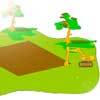New House

Age 7 to 11 Challenge Level:

In this investigation, you must try to make houses using cubes. If the base must not spill over 4 squares and you have 7 cubes which stand for 7 rooms, what different designs can you come up with?New MMP Website

Details of new MMP website, April 2013.New to You?

Age 5 to 11New to You?

Age 5 to 11

We hope the new ideas and situations in these activities will make you curious to know more.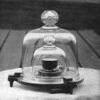New Units for Old

Age 16 to 18 Challenge Level:

Can you match up the entries from this table of units?Newspaper Sheets

Age 14 to 16 Short Challenge Level:

From only the page numbers on one sheet of newspaper, can you work out how many sheets there are altogether?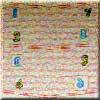Newspapers

Age 7 to 11 Challenge Level:

When newspaper pages get separated at home we have to try to sort them out and get things in the correct order. How many ways can we arrange these pages so that the numbering may be different?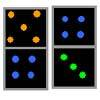Next Domino

Age 5 to 7 Challenge Level:

Which comes next in each pattern of dominoes?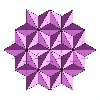Next Number

Age 7 to 11 Short Challenge Level:

Find the next number in this pattern: 3, 7, 19, 55 ...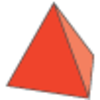Next Size Up

Age 7 to 11 Challenge Level:

The challenge for you is to make a string of six (or more!) graded cubes.Next-door Numbers

Age 5 to 7 Challenge Level:

Florence, Ethan and Alma have each added together two 'next-door' numbers. What is the same about their answers?Nice or Nasty

Age 7 to 14 Challenge Level:

There are nasty versions of this dice game but we'll start with the nice ones...Nice or Nasty for Two

Age 7 to 14 Challenge Level:

Some Games That May Be Nice or Nasty for an adult and child. Use your knowledge of place value to beat your opponent.Nicely Similar

Age 14 to 16 Challenge Level:

If the hypotenuse (base) length is 100cm and if an extra line splits the base into 36cm and 64cm parts, what were the side lengths for the original right-angled triangle?